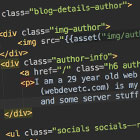## PHP: How does PHP compare objects?

If two objects are compared with `==`, then it will check if:

1) - they are two instances of the same type of class

2) - they have the same properties

3) - and those properties have the same values

`\$a = new SomeClass();\$a->something = 123; \$b = new SomeClass();\$b->something = 123; return \$a == \$b; // true`

If two objects are compared with `===`, then PHP will return true only if both variables are pointing to the exact same object (the same instance of the object). Remember that objects are always passed by value.

For example, the following will return true:

`\$a = new SomeClass();\$a->something = 123;\$b = \$a; // \$a and \$b point to the exact same instance return \$a === \$b; // true`

However, the following (using `===`) will return false. If the check was with `==` then it would return true, as the class type/properties/values are the same

`\$a = new SomeClass();\$a->something = 123; \$b = new SomeClass(); // different instance\$b->something = 123;  // same values return \$a === \$b; // false`webdevetc

I am a 29 year old backend web developer from London, mostly focusing on PHP and Laravel lately. This (webdevetc.com) is my blog where I write about some web development topics (PHP, Laravel, Javascript, and some server stuff). contact me here.